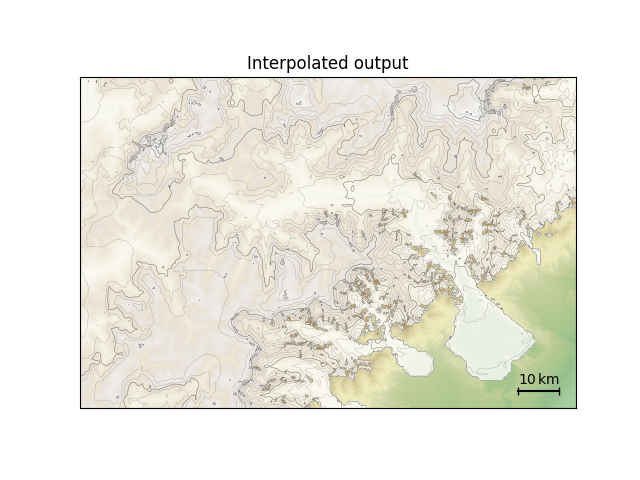# Interpolated with surface topo#

Demonstrate interpolating two-dimensional model output when surface topography, rather than bedrock topography, is present in the original data. The result is the same as in the interpolated output example.```downloading https://raw.githubusercontent.com/juseg/hyoga-data/main/pism/pism.alps.vis.refined.nc...
```

```import matplotlib.pyplot as plt
import hyoga

# initialize figure
ax = plt.subplot()

# open demo data
with hyoga.open.example('pism.alps.out.2d.nc') as ds:

# compute surface altitude and remove bedrock altitude
ds['usurf'] = ds.hyoga.getvar('surface_altitude')
ds = ds.drop('topg')

# compute isostatic adjustment from a reference input topography
ds = ds.hyoga.assign_isostasy(hyoga.open.example('pism.alps.in.boot.nc'))

# perform the actual interpolation
ds = ds.hyoga.interp(hyoga.open.example('pism.alps.vis.refined.nc'))

# plot model output
ds.hyoga.plot.bedrock_altitude(ax=ax, cmap='Topographic', center=False)
ds.hyoga.plot.surface_altitude_contours(ax=ax)
ds.hyoga.plot.ice_margin(ax=ax, facecolor='w')